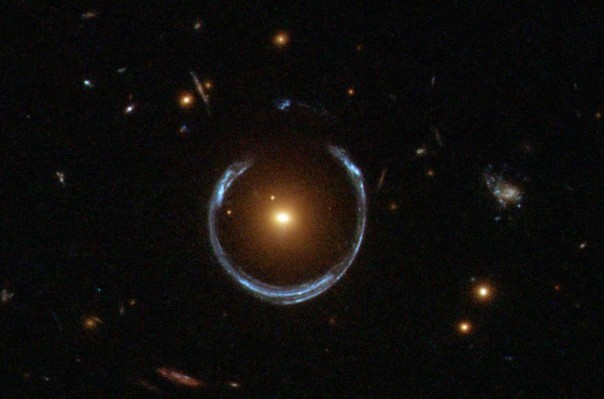# How to Look at the Back of Your Head Using a Black HoleA black hole is created when matter is compressed into such a small volume that the gravitational field that it creates becomes so strong that light cannot escape. The size that an object needs to be compressed to is called the Schwarzschild radius, and is given by a simple equation:$r_S = \frac{2GM}{c^2}$

where$r$ is the Schwarzschild radius,$G$ is the constant of universal gravitation,$M$ is the mass of the object, and$c$ is the speed of light. There is no lower limit to the mass$M$ involved: even the Earth could become a black hole if it were compressed into a ball less than eighteen millimetres across.

A black hole has no physical size (it is a singularity) but the “edge” of a black hole is usually taken to be its event horizon: the point beyond which even light cannot escape. The distance of the event horizon from “centre” of a black hole is equal to the black hole’s Schwarzschild radius. If some familiar objects from our solar system were to become black holes, their event horizons would be as follows:

 Object Event Horizon Earth 8.87 mm Saturn 84.4 cm Jupiter 2.82 m Sun 2.95 km

Objects will orbit a black hole just as they would orbit any other object with mass. If our Sun were to spontaneously become a black hole, the orbit of the planets in the solar system would be unaffected.

The closer an orbiting object is to the object it is orbiting, the faster it has to be travelling. That sentence is a bit difficult to understand, so I’ll explain it with an example: moving the Earth closer and closer to the Sun.

 Distance Sun to Earth /AU Required orbital speed /km/s 1.00 29.8 0.75 34.4 0.50 42.2 0.25 59.6

Eventually we get so close to the Sun that the speed required to remain in orbit becomes equal to the speed of light. This means that only photons (which travel at the speed of light) could orbit, and in fact, would orbit. Any photons that bounced off the back of your head would travel in a circular orbit around the black hole and end up at your eyes – you’d be able to look directly at the back of your head without using mirrors!

This effect is called a photon sphere, and can definitely exist around black holes.* It is also possible that a neutron star could be so compact that a photon sphere could exist, but this has never been observed.

* Around a rotating black hole there would be two photon spheres due to frame dragging: one would be closer, with the direction of orbit being the same as the direction of rotation, and one would be further away, with the direction of orbit being opposite to the direction of rotation.